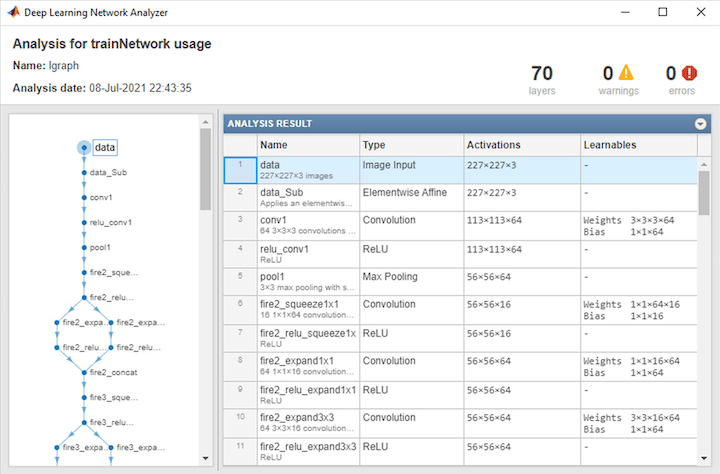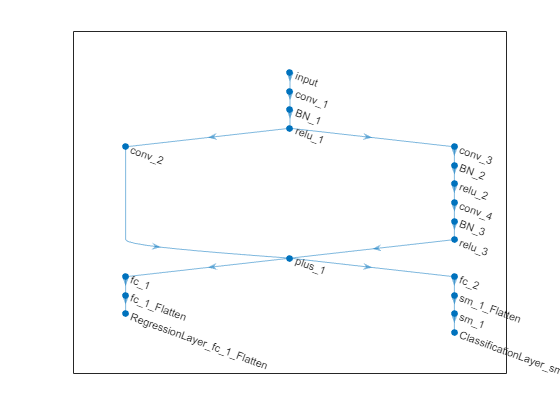# importONNXLayers

Import layers from ONNX network

## Syntax

``layers = importONNXLayers(modelfile)``
``layers = importONNXLayers(modelfile,Name,Value)``

## Description

example

````layers = importONNXLayers(modelfile)` imports the layers of an ONNX™ (Open Neural Network Exchange) network from the file `modelfile`. You can train the imported layers on a new data set or assemble the layers into a network ready for prediction.This function requires the Deep Learning Toolbox™ Converter for ONNX Model Format support package. If this support package is not installed, then the function provides a download link.```

example

````layers = importONNXLayers(modelfile,Name,Value)` imports the layers from an ONNX network with additional options specified by one or more name-value pair arguments.For example, `importONNXLayers(modelfile,'ImportWeights',false)` imports the network architecture without weights from the file `modelfile`.```

## Examples

collapse all

Download and install the Deep Learning Toolbox Converter for ONNX Model Format support package.

Type `importONNXLayers` at the command line.

`importONNXLayers`

If Deep Learning Toolbox Converter for ONNX Model Format is not installed, then the function provides a link to the required support package in the Add-On Explorer. To install the support package, click the link, and then click . Check that the installation is successful by importing the network from the model file `'cifarResNet.onnx'` at the command line. If the support package is installed, then the function returns a `DAGNetwork` object.

```modelfile = 'cifarResNet.onnx'; layers = importONNXLayers(modelfile,'OutputLayerType','classification')```
```layers = LayerGraph with properties: Layers: [77×1 nnet.cnn.layer.Layer] Connections: [85×2 table]```

Import the architecture and weights of a residual neural network trained on the CIFAR-10 data set. Specify the file containing the ONNX network and the type of the output layer to add to the imported network.

```modelfile = 'cifarResNet.onnx'; lgraph = importONNXLayers(modelfile, ... 'OutputLayerType','classification', ... 'ImportWeights',true)```
```lgraph = LayerGraph with properties: Layers: [77×1 nnet.cnn.layer.Layer] Connections: [85×2 table] InputNames: {'Input_input'} OutputNames: {'ClassificationLayer_softmax'} ```

Analyze the imported network architecture.

`analyzeNetwork(lgraph)`Import an ONNX network that has multiple outputs by using `importONNXLayers`. The function inserts placeholder layers for the outputs. After importing, you can find and replace the placeholder layers by using `findPlaceholderLayers` and `replaceLayer`, respectively.

Specify the network file from which to import layers and weights.

`modelfile = 'digitsMIMO.onnx';`

The network in `digitsMIMO.onnx` has two output layers: one classification layer to classify digits and one regression layer to compute the mean squared error for the predicted angles of the digits. Import the layers and weights from `modelfile`.

`layers = importONNXLayers('digitsMIMO.onnx','ImportWeights',true)`
```Warning: ONNX network has multiple outputs. importONNXLayers inserts placeholder layers for the outputs. Find and replace the layers by using findPlaceholderLayers and replaceLayer, respectively. ```
```layers = LayerGraph with properties: Layers: [19×1 nnet.cnn.layer.Layer] Connections: [19×2 table] InputNames: {'Input_input'} OutputNames: {1×0 cell} ```

`importONNXLayers` displays a warning and inserts placeholder layers for the output layers.

Plot the layer graph using `plot`.

`plot(layers)`The layer graph has two output layers: `Output_fc_1_Flatten` and `Output_sm_1`. These two layers are the placeholders for the outputs. You can check the placeholder layers by viewing the `Layers` property or by using the `findPlaceholderLayers` function.

`layers.Layers`
```ans = 19×1 Layer array with layers: 1 'Input_input' Image Input 28×28×1 images 2 'conv_1' Convolution 16 5×5×1 convolutions with stride [1 1] and padding [2 2 2 2] 3 'BN_1' Batch Normalization Batch normalization with 16 channels 4 'relu_1' ReLU ReLU 5 'conv_2' Convolution 32 1×1×16 convolutions with stride [2 2] and padding [0 0 0 0] 6 'conv_3' Convolution 32 3×3×16 convolutions with stride [2 2] and padding [1 1 1 1] 7 'BN_2' Batch Normalization Batch normalization with 32 channels 8 'relu_2' ReLU ReLU 9 'conv_4' Convolution 32 3×3×32 convolutions with stride [1 1] and padding [1 1 1 1] 10 'BN_3' Batch Normalization Batch normalization with 32 channels 11 'relu_3' ReLU ReLU 12 'plus_1' Addition Element-wise addition of 2 inputs 13 'fc_1' Convolution 1 14×14×32 convolutions with stride [1 1] and padding [0 0 0 0] 14 'fc_2' Convolution 10 14×14×32 convolutions with stride [1 1] and padding [0 0 0 0] 15 'sm_1_Flatten' ONNX Flatten Flatten activations into 1-D assuming C-style (row-major) order 16 'sm_1' Softmax softmax 17 'Output_sm_1' PLACEHOLDER LAYER Placeholder for 'Output' ONNX operator 18 'fc_1_Flatten' ONNX Flatten Flatten activations into 1-D assuming C-style (row-major) order 19 'Output_fc_1_Flatten' PLACEHOLDER LAYER Placeholder for 'Output' ONNX operator ```
`placeholderLayers = findPlaceholderLayers(layers)`
```placeholderLayers = 2×1 PlaceholderOutputLayer array with layers: 1 'Output_sm_1' PLACEHOLDER LAYER Placeholder for 'Output' ONNX operator 2 'Output_fc_1_Flatten' PLACEHOLDER LAYER Placeholder for 'Output' ONNX operator ```

Create output layers to replace the placeholder layers. First, create a classification layer with the name `Output_sm_1`. Specify the classes of the output layer as `0`, `1`, ..., `9`. If you do not specify the classes, then the software automatically sets them to `1`, `2`, ..., `N`, where `N` is the number of classes.

`output1 = classificationLayer('Name','Output_sm_1','Classes',string(0:9)); `

Create a regression layer with the name `Output_fc_1_Flatten`.

`output2 = regressionLayer('Name','Output_fc_1_Flatten'); `

Replace the placeholder layers with `output1` and `output2` using `replaceLayer`.

```layers = replaceLayer(layers,'Output_sm_1',output1); layers = replaceLayer(layers,'Output_fc_1_Flatten',output2);```

Display the `Layers` property of the layer graph to confirm the replacement.

`layers.Layers`
```ans = 19×1 Layer array with layers: 1 'Input_input' Image Input 28×28×1 images 2 'conv_1' Convolution 16 5×5×1 convolutions with stride [1 1] and padding [2 2 2 2] 3 'BN_1' Batch Normalization Batch normalization with 16 channels 4 'relu_1' ReLU ReLU 5 'conv_2' Convolution 32 1×1×16 convolutions with stride [2 2] and padding [0 0 0 0] 6 'conv_3' Convolution 32 3×3×16 convolutions with stride [2 2] and padding [1 1 1 1] 7 'BN_2' Batch Normalization Batch normalization with 32 channels 8 'relu_2' ReLU ReLU 9 'conv_4' Convolution 32 3×3×32 convolutions with stride [1 1] and padding [1 1 1 1] 10 'BN_3' Batch Normalization Batch normalization with 32 channels 11 'relu_3' ReLU ReLU 12 'plus_1' Addition Element-wise addition of 2 inputs 13 'fc_1' Convolution 1 14×14×32 convolutions with stride [1 1] and padding [0 0 0 0] 14 'fc_2' Convolution 10 14×14×32 convolutions with stride [1 1] and padding [0 0 0 0] 15 'sm_1_Flatten' ONNX Flatten Flatten activations into 1-D assuming C-style (row-major) order 16 'sm_1' Softmax softmax 17 'Output_sm_1' Classification Output crossentropyex with '0' and 9 other classes 18 'fc_1_Flatten' ONNX Flatten Flatten activations into 1-D assuming C-style (row-major) order 19 'Output_fc_1_Flatten' Regression Output mean-squared-error ```

Assemble the layer graph using `assembleNetwork`. The function returns a `DAGNetwork` object that is ready to use for prediction.

`assembledNet = assembleNetwork(layers)`
```assembledNet = DAGNetwork with properties: Layers: [19×1 nnet.cnn.layer.Layer] Connections: [19×2 table] InputNames: {'Input_input'} OutputNames: {'Output_sm_1' 'Output_fc_1_Flatten'} ```

## Input Arguments

collapse all

Name of ONNX model file containing the network, specified as a character vector or a string scalar. The file must be in the current folder, in a folder on the MATLAB® path, or you must include a full or relative path to the file.

Example: `'cifarResNet.onnx'`

### Name-Value Pair Arguments

Specify optional comma-separated pairs of `Name,Value` arguments. `Name` is the argument name and `Value` is the corresponding value. `Name` must appear inside quotes. You can specify several name and value pair arguments in any order as `Name1,Value1,...,NameN,ValueN`.

Example: `importONNXLayers(modelfile,'OutputLayerType','classification')` imports the network layers from `modelfile` and adds an output layer for a classification output layer at the end of the imported layers.

Type of the output layer that the function appends to the end of the imported network architecture, specified as `'classification'`, `'regression'`, or `'pixelclassification'`. Using `'pixelclassification'` appends a `pixelClassificationLayer` (Computer Vision Toolbox) object (requires Computer Vision Toolbox™).

If a network in `modelfile` has multiple outputs, then you cannot specify the output layer types using this argument. `importONNXLayers` inserts placeholder layers for the outputs. After importing, you can find and replace the placeholder layers by using `findPlaceholderLayers` and `replaceLayer`, respectively.

Example: `'OutputLayerType','regression'`

Indicator to import weights as well as the network architecture, specified as either `false` or `true`.

Example: `'ImportWeights',true`

Data Types: `logical`

## Output Arguments

collapse all

Network architecture, returned as a `LayerGraph` object.

## Limitations

• `importONNXLayers` supports ONNX versions as follows:

• The function supports ONNX intermediate representation version 6.

• The function fully supports ONNX operator sets 6, 7, 8, and 9.

• The function offers limited support for ONNX operator sets 10 and 11.

Note

If you import an exported network, layers of the reimported network might differ from the original network and might not be supported.

collapse all

### Supported ONNX Layers

`importONNXLayers` supports the following ONNX layers, with some limitations:

ONNX LayerDeep Learning Toolbox Layer

`Add`

`additionLayer` or `nnet.onnx.layer.ElementwiseAffineLayer`

`AveragePool`

`averagePooling2dLayer`

`BatchNormalization`

`batchNormalizationLayer`

`Concat`

`concatenationLayer`

`Constant`

None (Imported as weights)

`Conv`*

`convolution2dLayer`

`ConvTranspose`

`transposedConv2dLayer`

`Dropout`

`dropoutLayer`

`Elu`

`eluLayer`

`Gemm`

`fullyConnectedLayer` if ONNX network is recurrent, otherwise `nnet.onnx.layer.FlattenLayer` followed by `convolution2dLayer`

`GlobalAveragePool`

`globalAveragePooling2dLayer`

`GlobalMaxPool`

`globalMaxPooling2dLayer`

`GRU`

`gruLayer`

`InstanceNormalization`

`groupNormalizationLayer` with `numGroups` specified as `"channel-wise"`

`LeakyRelu`

`leakyReluLayer`

`LRN`

`CrossChannelNormalizationLayer`

`LSTM`

`lstmLayer` or `bilstmLayer`

`MatMul`

`fullyConnectedLayer` if ONNX network is recurrent, otherwise `convolution2dLayer`

`MaxPool`

`maxPooling2dLayer`

`Mul`

`multiplicationLayer`

`Relu`

`reluLayer` or `clippedReluLayer`

`Sigmoid`

`sigmoidLayer`

`Softmax`

`softmaxLayer`

`Sum`

`additionLayer`

`Tanh`

`tanhLayer`

*If the `pads` attribute of the `Conv` operator is a vector with only two elements `[p1,p2]`, `importONNXLayers` imports `Conv` as a `convolution2dLayer` with the name-value argument `'Padding'` specified as `[p1,p2,p1,p2]`.

ONNX LayerONNX Importer Custom Layer

`Clip`

`nnet.onnx.layer.ClipLayer`

`Div`

`nnet.onnx.layer.ElementwiseAffineLayer`

`Flatten`

`nnet.onnx.layer.FlattenLayer` or `nnet.onnx.layer.Flatten3dLayer`

`Identity`

`nnet.onnx.layer.IdentityLayer`

`ImageScaler`

`nnet.onnx.layer.ElementwiseAffineLayer`

`PRelu`

`nnet.onnx.layer.PReluLayer`

`Reshape`

`nnet.onnx.layer.FlattenLayer`

`Sub`

`nnet.onnx.layer.ElementwiseAffineLayer`
ONNX LayerImage Processing Toolbox™
`DepthToSpace``depthToSpace2dLayer` (Image Processing Toolbox)
`Resize``resize2dLayer` (Image Processing Toolbox) or `resize3dLayer` (Image Processing Toolbox)
`SpaceToDepth``spaceToDepthLayer` (Image Processing Toolbox)
`Upsample``resize2dLayer` (Image Processing Toolbox) or `resize3dLayer` (Image Processing Toolbox)

## Tips

 Open Neural Network Exchange. https://github.com/onnx/.

 ONNX. https://onnx.ai/.